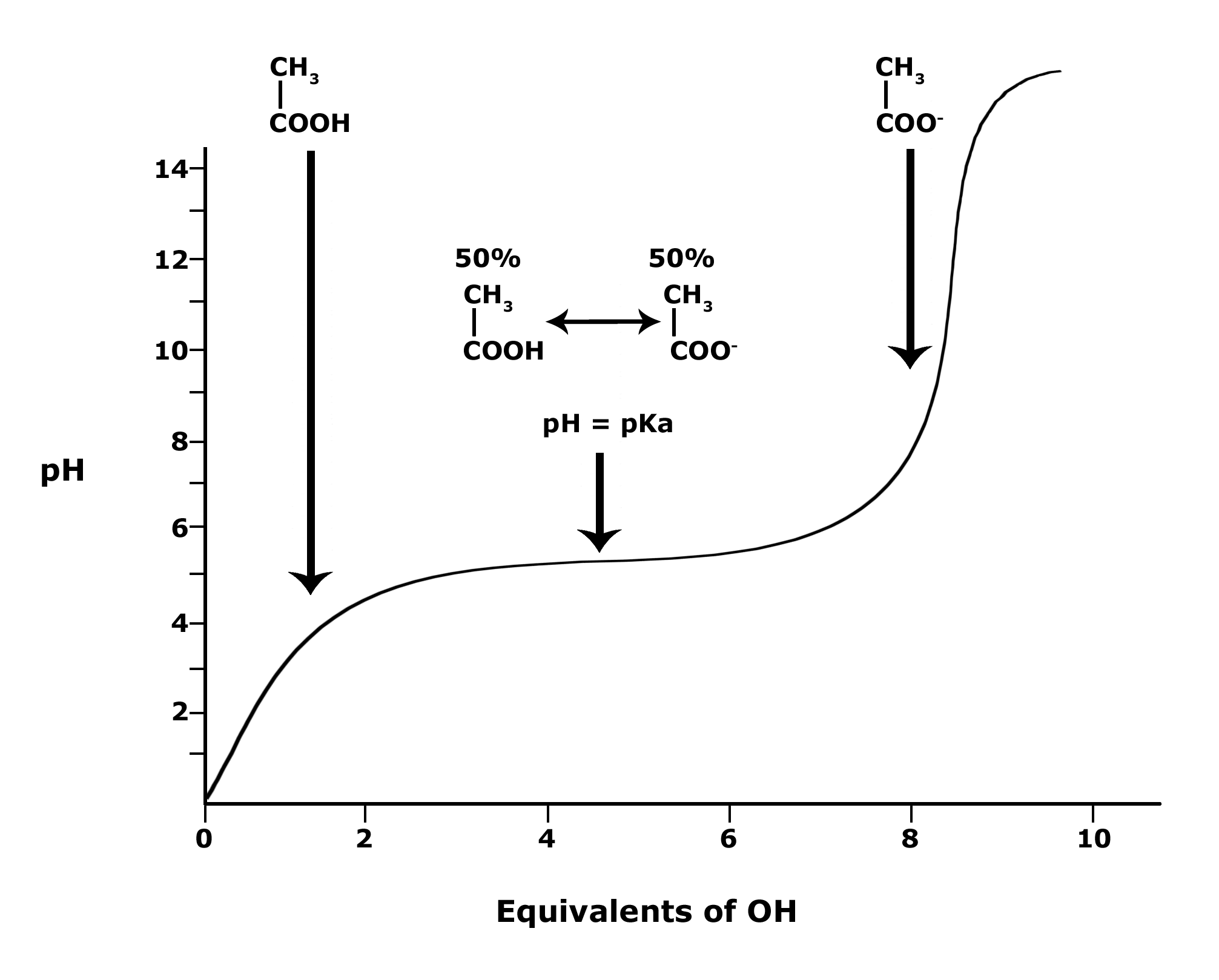# 2020_Spring_Bis2a_Facciotti_Lecture_05

### Learning Objectives Associated with 2020_Spring_Bis2a_Facciotti_Lecture_05

• Define the acid dissociation constant, Ka
• Define pKa
• Explain the relationship between pKa and pH and apply these concepts to predict the protonation state of a functional group at different pH values.

## pKapKa is defined as the negative log10 of the dissociation constant of an acid, its Ka. Therefore, the pKa is a quantitative measure of how easy or how readily the acid gives up its proton [H+] in solution and thus a measure of the "strength" of the acid. Strong acids have a small pKa, weak acids have a larger pKa.

The most common acid we will talk about in BIS2A is the carboxylic acid functional group. These acids are weak acids, meaning that they only partially dissociate (into H+ cations and RCOO- anions) in a neutral solution. HCL (hydrogen chloride) is a common strong acid, meaning that it will fully dissociate into H+ and Cl-.

Note that the key difference in the figure below between a strong acid or base and a weak acid or base is the single arrow (strong) versus a double arrow (weak). In the case of the single arrow, you can interpret that by imagining that nearly all reactants have been converted into products. It is difficult for the reaction to reverse backwards to a state where the protons are again associated with the molecule there were associated with before. With a weak acid or base, the double-sided arrow can be interpreted by picturing a reaction in which:

1. both forms of the conjugate acid or base (that is what we call the molecule that "holds" the proton - i.e. CH3OOH and CH3OO-, respectively in the figure) are present at the same time and
2. the ratio of those two quantities can change easily by moving the reaction in either direction.Figure 1. An example of strong acids and strong bases in their protonation and deprotonation states. The value of their pKa is shown on the left. Attribution: Marc T. Facciotti.

In Bis2A you we ask you to relate pH and pKa to each other when discussing the protonation state of an acid or base, for example, in amino acids. How can we use the information given in this module to answer the question: Will the functional groups on the amino acid Glutamate be protonated or deprotonated at a pH of 2, at a pH of 8, at a pH of 11?

To answer this question, we need to create a relationship between pH and pKa. The relationship between pKa and pH is mathematically represented by Henderson-Hasselbach equation shown below, where [A-] represents the deprotonated form of the acid and [HA] represents the protonated form of the acid.Figure 2. The Henderson-Hasselbach equation

A solution to this equation is obtained by setting pH = pKa. Here, log([A-] / [HA]) = 0, and [A-] / [HA] = 1. This means that when the pH is equal to the pKa there are equal amounts of protonated and deprotonated forms of the acid. For example, if the pKa of the acid is 4.75, at a pH of 4.75 that acid will exist as 50% protonated and 50% deprotonated. This also means that as the pH rises, more of the acid will convert to the deprotonated state and at some point the pH will be so high that most of the acid will exist in the deprotonated state.Figure 3. This graph depicts the protonation state of acetic acid as the pH changes. At a pH below the pKa, the acid is protonated. At a pH above the pKa the acid is deprotonated. If the pH equals the pKa, the acid is 50% protonated and 50% deprotonated. Attribution: Ivy Jose

In BIS2A, we will look at the protonation state and deprotonation state of amino acids. Amino acids contain multiple functional groups that can be acids or bases. Therefore, their protonation/deprotonation status can be more complicated. Below is the relationship between the pH and pKa of the amino acid Glutamic Acid. In this graph we can ask: Will the functional groups on the amino acid Glutamate be protonated or deprotonated at a pH of 2, at a pH of 8, at a pH of 11?Figure 4. This graph depicts the protonation state of glutamate as the pH changes. At a pH below the pKa for each functional group on the amino acid, the functional group is protonated. At a pH above the pKa for the functional group it is deprotonated. If the pH equals the pKa, the functional group is 50% protonated and 50% deprotonated.
Possible NB DiscussionPoint